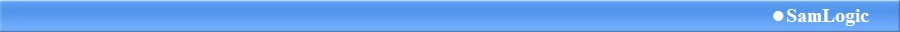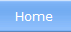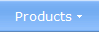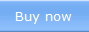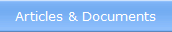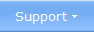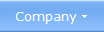Check if the operating system is 32 bit or 64 bit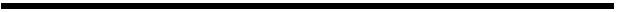Another condition that we have added to Visual Installer's scripting language is to test if the operating system that the setup program is run in is 32 bit or 64 bit. This is useful, for example, if you have both a 32 bit and a 64 bit installation package that you want to run from Visual Installer, but you want to make sure that the 64 bit installation package is only run in a 64 bit operating system, and the 32 bit installation package is only run in a 32 bit operating system. By using the new condition you can choose correct bitness for the installation package.

To test if the operating system is a 32 bit system you can use the following syntax:

 IF OSBIT=32     Do some stuff END IF

To test if the operating system is a 64 bit system you can use the following syntax:

 IF OSBIT=64     Do some stuff END IF

The example below shows how to combine the IF OSBIT=64 condition with the MSIEXEC command to make sure that a 64 bit installation package is only run in a 64 bit operating system: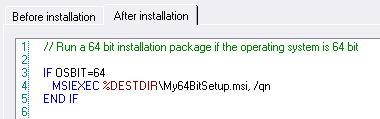< Go back